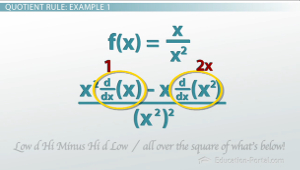# When to Use the Quotient Rule for Differentiation

An error occurred trying to load this video.

Try refreshing the page, or contact customer support.

Coming up next: Understanding Higher Order Derivatives Using Graphs

### You're on a roll. Keep up the good work!

Replay
Your next lesson will play in 10 seconds
• 0:06 Introduction to the…
• 1:18 Understanding the…
• 4:09 Quotient Rule Examples
• 7:29 Lesson Summary

Want to watch this again later?

Timeline
Autoplay
Autoplay
Speed

#### Recommended Lessons and Courses for You

Lesson Transcript
Instructor: Eric Garneau
Lo D Hi minus Hi D Lo, all over the square of what's below! Learn the quotient rule chant for differentiating functions that take the form of fractions in this lesson.

## Introduction to the Quotient Rule

The quotient rule is the last of the main rules for calculating derivatives, and it primarily deals with what happens if you have a function divided by another function and you want to take the derivative of that. So let's start with f(x) = x / x^2. What is the derivative of f(x)? Is it just the top? Is it the bottom? Is it the derivative of the top times the derivative of the bottom? What is it? Well you could simplify this into 1 / x, and then f`(x) would then be -1 / x^2, because 1 / x is the same as x^-1. Then we just use our power rule. But is there a way to find that without simplifying? You won't be able to simplify in every case. Think about sin(x) / x, or (x^2 + ln(x)) / cos(x). What about those cases? You need to know the quotient rule.## Understanding the Quotient Rule

Let's say that you have y=u / v, where both u and v depend on x. Then you want to find dy/dx, or d/dx(u / v). There are two ways to find that. One is to use the power rule, then the product rule, then the chain rule. First you redefine u / v as uv^-1. Then you're going to differentiate; y` is the derivative of uv^-1. You need to use the product rule. So you've got y`= u(d/dx)v^-1 + v^-1(d/dx)u. Then we need to use the chain rule to differentiate v^-1, so y`= u(-1(1 / v^2)v`) + (v^-1)u`. I can rewrite this as y`= (u` / v) - (uv` / v^2). I can put all of this together by multiplying the left term by (v / v), so y` ends up being (vu` - uv`) / v^2. And that's what happens if you try to use the power rule, the product rule and the chain rule to differentiate u/v.

To unlock this lesson you must be a Study.com Member.

### Register to view this lesson

Are you a student or a teacher?

### Unlock Your Education

#### See for yourself why 30 million people use Study.com

##### Become a Study.com member and start learning now.
Back
What teachers are saying about Study.com

### Earning College Credit

Did you know… We have over 160 college courses that prepare you to earn credit by exam that is accepted by over 1,500 colleges and universities. You can test out of the first two years of college and save thousands off your degree. Anyone can earn credit-by-exam regardless of age or education level.